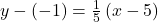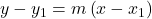## Find a Point-Slope equation for a line containing the given point and having the given slope. (5, -1), m =1/5

Question

Find a Point-Slope equation for a line containing the given point and having the given slope.

(5, -1), m =1/5

in progress 0
1 week 2021-09-04T18:33:48+00:00 1 Answers 0 views 0

The point-slope equation for a line is:Step-by-step explanation:

Given

• The point = (5, -1)
• m = 1/5

We know that the point-slope form iswhere m is the slope of the line

substituting the values m = 1/5 and the point (5, -1)Thus, the point-slope equation for a line is: